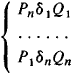# Normal Algorithm

The following article is from The Great Soviet Encyclopedia (1979). It might be outdated or ideologically biased.

## Normal Algorithm

one of the modern refinements of the concept of algorithm that has become widespread in constructive mathematics. The term was proposed in 1950 by A. A. Markov, who was the first to systematically and rigorously construct a general theory of algorithms based on this refinement. Normal algorithms are equivalent to partial recursive functions and, consequently, to Turing machines.

The concept of normal algorithm has been specially adapted for the purpose of devising algorithms applicable to words in various alphabets. In this case, in mathematics, an alphabet is understood to mean any finite set of strictly distinguishable graphic symbols (letters), and a word in an alphabet means an arbitrary finite sequence of letters of the alphabet. A sequence that contains no letters whatever also is considered to be a word in the given alphabet (the “empty” word). For example, the sequences “ooaam,” “major,” and “gamma” are words in the English alphabet and also in the six-letter alphabet {m, a, j, o, r, g}. The operation of “substitution for the first occurrence” is an elementary act of transforming words in the algorithmic processes defined by the normal algorithm. Let P, Q, and R be words in some alphabet. The word ∑(R, P, Q), which is obtained in the manner described below, is the result of the substitution of Q for the first occurrence of P in R. If P occurs in R, that is, if R is representable in the form S1PS2, then the representation having the shortest word S1 is sought among such representations, and it is assumed that ∑(R, P, Q) = S1QS2. If, however, P does not occur in R, then ∑(R, P, Q) = R. Thus, 1 (gamma, a, e) = gemma. In order to define a normal algorithm U, it is necessary to establish a certain alphabet A that does not contain the symbols “→,” and “·” and an ordered list of expressions of the type PQ (a simple production) or P · Q (a terminal production), where P and Q are words in A. The productions are commonly written in a vertical column in sequence and joined by a brace on the left. The resultant figure is called the algorithm scheme of the normal algorithm. Both the initial data and the results of the operation of the normal algorithm U are words in A; the normal algorithm U itself is said to be a normal algorithm in alphabet A. The process of applying to the word R the normal algorithm U having an algorithm scheme of the formwhere δi(1 ≤ in) designates “→,” or “→, ·”, can be described as follows. We seek the smallest i for which Pi occurs in R. If none of the Pi occur in R, then the operation of U is terminated and R is considered to be the result. If the required i is found, then R is transformed into the word Σ(R, Pi, Qi). If the production Piδi Qi that is used is terminal (δi = → ·), then the operation of U terminates and Σ(R, Pi, Qi) is considered to be the result. If, however, the production Pi, QiP P,i is simple, then the procedure described above is repeated, with Σ(R, Pi, Qi) in place of R.

Example. The natural numbers may be considered as words in the alphabet {0, 1} of the form 0, 01, 011, … Normal algorithms in this alphabet with algorithm schemes {0→ · 01 and {1 →, transform each natural number n into n + 1 and 0, respectively.

The set of all normal algorithms is closed with respect to known methods of combining algorithms. For example, for any two normal algorithms U and ℬ, we can construct a normal algorithm C that is a composite of U and ℬ; that is, C satisfies the following intuitive algorithm: “first perform algorithm and then apply ℬ to the result.”

The relation between intuitive algorithms and normal algorithms is described by the normalization principle introduced by Markov: any algorithm that transforms words in a given alphabet A into words in the same alphabet can be realized by means of a normal algorithm in some extension of A. (We can readily point out very simple algorithms in A that cannot be realized by a normal algorithm in A; on the other hand, we can always restrict ourselves to a two-letter, or even a one-letter, extension of A.) The normalization principle is equivalent to Church’s thesis and, like Church’s thesis, is not subject to proof because of the inexactness of the intuitive concept of algorithm.

### REFERENCES

Markov, A. A. Teoriia algorifmov. Moscow-Leningrad, 1954. (Tr. Matematicheskogo instituta AN SSSR, vol. 42.)
Mendelson, E. Vvedenie v matematicheskuiu logiku. Moscow, 1971. (Translated from English.)

B. A. KUSHNER